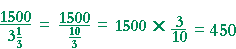simultaneous equations

Section 2

Back to Section 1:  Examples

Problem 1.   A woman is now 30 years older than her son.  15 years ago, she was twice as old.  What are the present ages of the woman and her son?

Do the problem yourself first!

Let x be the present age of the woman.

Let y be the present age of her son.

Here are the equations:

 1) Present age of the woman in relation to her son: x = y + 30. 2) 15 years ago: x − 15 = 2(y − 15).

To eliminate x, simply substitute equation 1) in equation 2), and solve for y.

You should find:  y = 45 years.

Therefore x, the age of the woman, is 75 years.

Problem 2.   A total of 925 tickets were sold for \$5,925.  If adult tickets cost \$7.50, and children's tickets cost \$3.00, how many tickets of each kind were sold?   (Compare Example 2.)

Let x be the number of adult tickets.   Let y be the number of childeren's tickets.

Here are the equations:

 1) Total number of tickets: x + y = 925 2) Total money collected: 7.5x + 3y = 5,925

In equation 2), make the coefficients into whole numbers by multiplying both sides of the equation by 10:

 1) x + y = 925 2') 75x + 30y = 59,250

To eliminate y, for example:

Multiply equation 1) by −30, and add.

The solution is:   x = 700,  y = 225.

Problem 3.   Mr. B. has \$20,000 to invest.  He invests part at 6%, the rest at 7%, and he earns \$1,280 interest.  How much did he invest at each rate?   (Compare Example 3.)

Let x be how much he inveted at 6%.   Let y be how much he inveted at 7%.

Here are the equations:

 1) Total investment: x + y = 20,000 2) Total interest: .06x + .07y = 1,280 2') 6x + 7y = 128,000

To eliminate x, for example, from equations 1) and 2'):

Multiply equation 1) by −6, and add.

The solution is:   x = \$12,000.  y = \$8,000.

Problem 4.   Edgar has 20 dimes and nickels, which together total \$1.40. How many of each does he have?   (Compare the Problem in Section 1.)

Let x be the number of dimes.   Let y be be the number of nickels.

Here are the equations:

 1) Total number of coins: x + y = 20 2) Total value: .10x + .05y = 1.4 2') 10x + 5y = 140

To eliminate x, for example, from equations 1) and 2'), multiply equation 1) by −10, and add.

The solution is:   x = 8 dimes.  y = 12 nickels.

Problem 5.   How many gallons of 20% alcohol solution  and how many of 50% alcohol solution must be mixed  to produce 9 gallons of 30% alcohol solution?   (Compare Example 4.)

(9 gallons of 30% alcohol solution = .3 × 9 = 2.7 gallons of pure alcohol.)

Let x be the number of gallons of 20% solution.   Let y be the number of gallons of 50% solution.

Here are the equations:

 1) Total number of gallons: x + y = 9 2) Total gallons of pure alcohol: .2x + .5y = 2.7 2') 2x + 5y = 27

To eliminate x, for example, from equations 1) and 2'), multiply equation 1) by −2, and add.

The solution is:   x = 6 gallons.  y = 3 gallons.

Problem 6.   15 gallons of 16% disenfectant solution is to be made from 20% and 14% solutions. How much of those solutions should be used?

(15 gallons of 16% solution = .16 × 15 = 2.4 gallons of pure disenfectant.)

Let x be the number of gallons of 20% solution.   Let y be the number of gallons of 14% solution.

Here are the equations:

 1) Total number of gallons: x + y = 15 2) Total gallons of pure disenfectant: .20x + .14y = 2.4 2') 20x + 14y = 240

To eliminate x, for example, from equations 1) and 2'), multiply equation 1) by −20, and add.

The solution is:   x = 5 gallons.  y = 10 gallons.

Problem 7.   It takes a boat 2 hours to travel 24 miles downstream  and 3 hours to travel 18 miles upstream. What is the speed of the boat in still water, and how fast is the current?   (Compare Example 6.)

Let x be the speed of the boat in still water.   Let y be the speed of the current.

Here are the equations:

 1) Downstream speed: x + y = 24 2 = 12 2) Upstream speed: x − y = 18 3 = 6

To eliminate y, simply add the equations.

The solution is:   x = 9 mph.  y = 3 mph.

Problem 8.   An airplane covers a distance of 1500 miles in 3 hours
 when it flies with the wind, and in 3 13 hours when it flies against the

wind.  What is the speed of the plane in still air?   (Compare Example 6.)

Let x be the speed of the plane in still air.  Let y be the speed of the wind.

Here are the equations:

 1) Speed with the wind: x + y = 1500   3 =  500 2) Speed against the wind : x − y =To eliminate y, simply add the equations.

The solution is:   x = 475 mph.

Back to Section 1